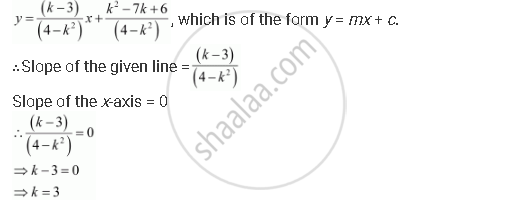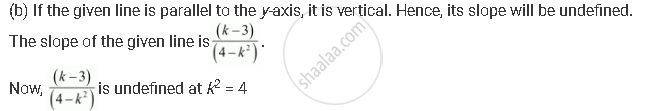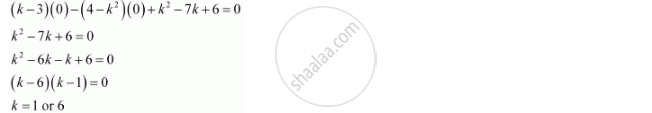CBSE (Arts) Class 11CBSE
Share

# Find the Values of K for Which the Line (K–3) X – (4 – K2) Y + K2 –7k + 6 = 0 is - CBSE (Arts) Class 11 - Mathematics

#### Question

Find the values of k for which the line (k–3) x – (4 – k2) y + k2 –7k + 6 = 0 is

(a) Parallel to the x-axis,

(b) Parallel to the y-axis,

(c) Passing through the origin.

#### Solution

The given equation of line is

(k – 3) x – (4 – k2y + k2 – 7k + 6 = 0 … (1)

(a) If the given line is parallel to the x-axis, then

Slope of the given line = Slope of the x-axis

The given line can be written as

(4 – k2y = (k – 3) x + k2 – 7k + 6 = 0Thus, if the given line is parallel to the x-axis, then the value of k is 3.k2 = 4

⇒ k = ±2

Thus, if the given line is parallel to the y-axis, then the value of k is ±2.

(c) If the given line is passing through the origin, then point (0, 0) satisfies the

given equation of line.Thus, if the given line is passing through the origin, then the value of k is either 1 or 6.

Is there an error in this question or solution?

#### Video TutorialsVIEW ALL 

Solution Find the Values of K for Which the Line (K–3) X – (4 – K2) Y + K2 –7k + 6 = 0 is Concept: Slope of a Line.
S